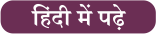Home   »   Important Days   »   Pi Approximation Day 2023

# Pi Approximation Day 2023: Date, Significance and History

Pi Approximation Day is observed on July 22 (22/7 in the day/month date format), since the fraction 22⁄7 is a common approximation of π, which is accurate to two decimal places and dates from Archimedes. A number of people celebrate March 14 as Pi Day as the date lines up in the numbers of the famous constant 3.14. However, such a format of writing dates is mostly followed in the US.

## History of Pi Approximation Day

Pi (π) was known and used ancient times approximated for almost 4000 years. The ancient Babylonians also calculated the area of the circle by taking three times the square of its radius which gave the value of pi equals to three. Archimedes of Syracuse (287–212 BC), one of the greatest mathematicians of the ancient world was the first person to do the calculation of π. Archimedes tried to show that the value π is between 3 1/7 and 3 10/71.

Pi Approximation day is a observed annually to celebrate the usage of the mathematical constant π (pi). Pie day is observed on March 14 and Pi Approximation Day is observed on July 22. Pie day was founded by Larry Shaw in the year 1988. On November 2019 United Nations designated Pi day as the International Day of Mathematics.

## What is Pi?

Pi is the ratio of the circumference of any circle to the diameter of that circle. It is represented as the Greek letter for p or Pi (π). Regardless of the size of the circle, the ratio is always equal to Pi, which is fractionally represented as 22/7 or 3.14 in the decimal form. According to the book, A History of Pi by Petr Beckmann, the Greek letter π was first used by William Jones in 1706. It is believed Jones used the letter as an abbreviation of the periphery, which turned into a standard mathematical notation nearly 30 years later.

In 1737, Leonhard Euler adopted and popularised the symbol of Pi. Georges Buffon, an 18th-century French mathematician, invented a way to calculate Pi based on probability.

## Following are few important facts about Pi

• The number π (/paɪ/) is a mathematical constant.
• It is defined as the ratio of a circle’s circumference to its diameter
• It is approximately equal to 3.14159.
• It is also referred to as Archimedes’ constant.
• Fractions such as 22/7 are commonly used to approximate it.
• Its decimal representation never ends and never settles into a permanently repeating pattern.
• It is known that π is a transcendental number:
• There is no zero in the first 31 digits of Pi
• At position 763 which is also known as Feynman Point, there are six nines in a row
• In 2015, Suresh Kumar Sharma, a former vegetable vendor from Jaipur, India now a memory coach, set a world record when he successfully recited more than 70,030 digits of pi.
• It took 17 hours to complete the memorization.
• Johann Lambert proved Pi is irrational in 1768
• As the exact value of pi cannot be calculated, we can never find the accurate area or circumference of a circle.
• The Welsh mathematician William Jones began using the symbol for pi in 1706.
• Lu Chao of China recited 67,890 digits of pi in the year 2005.

Find More Important Days Here